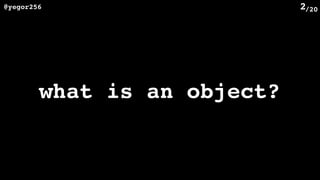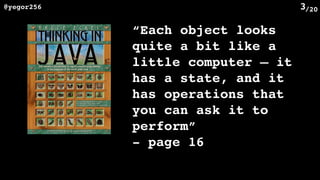Successfully reported this slideshow.

# How Immutability Helps in OOP

1

Share×
1 of 20
1 of 20

# How Immutability Helps in OOP

1

Share

JEEConf 2016; Kiev, Ukraine; 21 May 2016. Video is here: https://www.youtube.com/watch?v=EnhRgXrHCC4 (in Russian with English subtitles).

JEEConf 2016; Kiev, Ukraine; 21 May 2016. Video is here: https://www.youtube.com/watch?v=EnhRgXrHCC4 (in Russian with English subtitles).

## More Related Content

### Related Books

Free with a 14 day trial from Scribd

See all

### Related Audiobooks

Free with a 14 day trial from Scribd

See all

### How Immutability Helps in OOP

1. 1. /20@yegor256 1 How Immutability  Helps in OOP? Yegor Bugayenko
2. 2. /20@yegor256 2 what is an object?
3. 3. /20@yegor256 3 “Each object looks quite a bit like a little computer — it has a state, and it has operations that you can ask it to perform” - page 16
4. 4. /20@yegor256 4 “An object is some memory that holds a value of some type” - page 40
5. 5. /20@yegor256 5 “A class is a collection of data fields that hold values and methods that operate on those values” - page 98
6. 6. /20@yegor256 6 “Objects may contain data, in the form of fields, often known as attributes; and code, in the form of procedures, often known as methods”
7. 7. /20@yegor256 7
8. 8. /20@yegor256 8 class Calculator { private int[] array; public void calculate(int a, int b) { this.array = new int[b - a]; for (int i = 0; i < this.array.length; ++i) { this.array[i] = a + i; } } public int[] numbers() { return this.array; } }
9. 9. /20@yegor256 9 Calculator c = new Calculator(); c.calculate(5, 15); int[] x = c.numbers();
10. 10. /20@yegor256 10 “who I am?” vs. “what I do?”
11. 11. /20@yegor256 11 class Range { private final int a; private final int b; Range(int start, int finish) { this.a = start; this.b = finish; } public int[] numbers() { int[] array = new int[this.b - this.a]; for (int i = 0; i < array.length; ++i) { array[i] = this.a + i; } return array; } }
12. 12. /20@yegor256 12 Range r = new Range(5, 15); int[] x = r.numbers();
13. 13. /20@yegor256 13 R range = new Range(5, 15); int[] x = range.numbers(); Calculator c = new Calculator(); c.calculate(5, 15); int[] x = c.numbers();
14. 14. /20@yegor256 14 R range = new OddOnly( new Range(5, 15) ); int[] x = range.numbers(); Calculator c = new Calculator(); c.setOddOnly(true); c.calculate(5, 15); int[] x = c.numbers();
15. 15. /20@yegor256 15 R range = new SquareAll( new OddOnly( new Range(5, 15) ) ); int[] x = range.numbers(); Calculator c = new Calculator(); c.setOddOnly(true); c.setSquareAll(true); c.calculate(5, 15); int[] x = c.numbers();
16. 16. /20@yegor256 16 R range = new Incremented( new Squared( new OddOnly( new Range(5, 15) ) ), 100 ); int[] x = range.numbers(); Calculator c = new Calculator(); c.setOddOnly(true); c.setSquared(true); c.setIncremented(100); c.calculate(5, 15); int[] x = c.numbers();
17. 17. /20@yegor256 17 R range = new Logged( new Incremented( new Squared( new OddOnly( new Range(5, 15) ) ), 100 ) ); int[] x = range.numbers(); Calculator c = new Calculator(); c.setOddOnly(true); c.setSquared(true); c.setIncremented(100); c.setLogged(true); c.calculate(5, 15); int[] x = c.numbers();
18. 18. /20@yegor256 18 R range = new Logged( new Incremented( new Squared( new OddOnly( new RangeFromFile( new File(“a.txt”) ) ) ), 100 ) ); int[] x = range.numbers(); Calculator c = new Calculator(); c.setFromFile(“a.txt”); //??? c.setOddOnly(true); c.setSquared(true); c.setIncremented(100); c.setLogged(true); c.calculate(5, 15); int[] x = c.numbers();
19. 19. /20@yegor256 19 what is an object?
20. 20. /20@yegor256 20 www.yegor256.com